﻿ 基于遗传算法的无人机航迹规划

# 基于遗传算法的无人机航迹规划UAV Route Planning and Obstacle Avoidance

Abstract: This project is designed to integrate technologies such as unmanned aerial vehicles, trajectory planning, distance measurement and obstacle avoidance, etc. The research and development have the ability to independently plan trajectories and realize the transformation from UAV remote control flight technology to intelligently autonomous trajectory flight technology to solve the complex intelligent technical problems of unmanned aerial vehicles under the threat cost (terrain, environment, etc.) to achieve flight planning and complete tasks without artificial control, autonomously plan trajectories, thereby improving the task completion rate and greatly reducing the task completion time.

1. 引言

Kiva Systems公司：其Raffaello教授的团队多次公开展示了，单翼到多翼无人机的稳定飞行，以及任务性自动规划飞行。

2. 研究设计的总体思路

2.1. 项目研究基本内容

1) 地面站：使用者通过地面站可以浏览和查询所连接的四轴飞行器的飞行高度，状态以及位置，接收飞行器的信号，并且还可以向四轴飞行器发送飞行的指令。

2) 四轴飞行器：注册并认证后的四轴飞行器可以在此系统中的地面站进行连接，接受地面站的信号信息，同时具有可以向地面站发送讯号以及相关信息的功能。

3) 通讯平台：连接地面站和四轴飞行器，为地面站与四轴飞行器之间讯号以及相关信息相互传输提供中间平台。

2.2. 项目研究技术路线

1) 在全局规划阶段，基于等效数字地形，建立数字高程地图，数字高程地图是用地形采样点的经纬度和标高组成的数值阵列表示，要用于地形仿真、任务规划和地形的三维显示等。

3) 本项目在C#的环境下，搭建地面站，满足硬件之上的操作系统部分，包含驱动、操作系统、应用接口，以及系统之上的应用软件部分的主要功能，有摇杆遥控、飞行数据监测、飞行姿态、飞行定位、航拍视频、数据存储、航点编辑、软件设置等。

4) 利用数字地图将三维地形上的路径寻优问题转化成状态空间最优解的搜索问题，并采用遗传算法搜索最优解，进行航迹规划。

5) 对遗传算法进行改进，对最优航迹路线进行平滑处理，实现航迹规划更加平稳，提高飞行器的安全性。

6) 对四轴无人机优化后的航迹进行仿真实验，与未经优化的航迹进行对比，最终实现飞行器稳定安全飞行。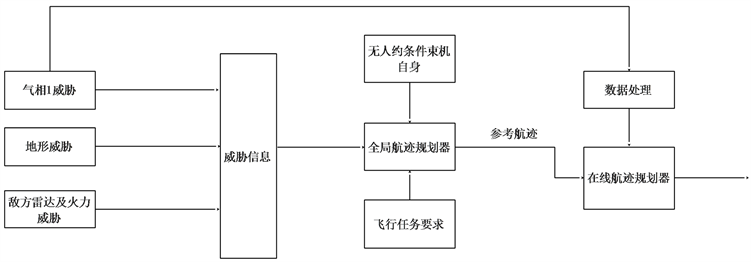Figure 1. Principle of UAV Route Planning

3. 项目研究主要原理

3.1. 地图的建立和种群编码

1) 不考虑障碍物高度问题，飞行器行走空间为二维平面空间；

2) 障碍物的大小、位置已知，并且不存在动态障碍物；

3) 在规划时可以把飞行器看作质点处理。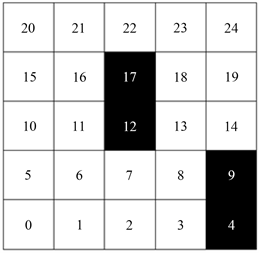$\begin{array}{l}x=\mathrm{int}\left(N/{G}_{size}\right)+1\\ y=N%Gsize+1\end{array}$

Gsize为每一行栅格数，int为取整操作。已为每一个栅格编好号码，因此可以用此编号来表示一条路径，比如起点为0终点为24的路径可以表示为(0, 6, 7, 8, 13, 19, 24)，在遗传算法中即为十进制编码。

3.2. 代码整体框架

3.3. 初始化种群

$D=\mathrm{max}\left\{abs\left({x}^{i+1}-{x}^{i}\right),abs\left({y}^{i+1}-{y}^{i}\right)\right\}$

$\begin{array}{l}{x}_{new}=\mathrm{int}\left(\frac{{x}_{i+1}+{x}_{i}}{2}\right)\\ {y}_{new}=\mathrm{int}\left(\frac{{y}_{i+1}+{y}_{i}}{2}\right)\end{array}$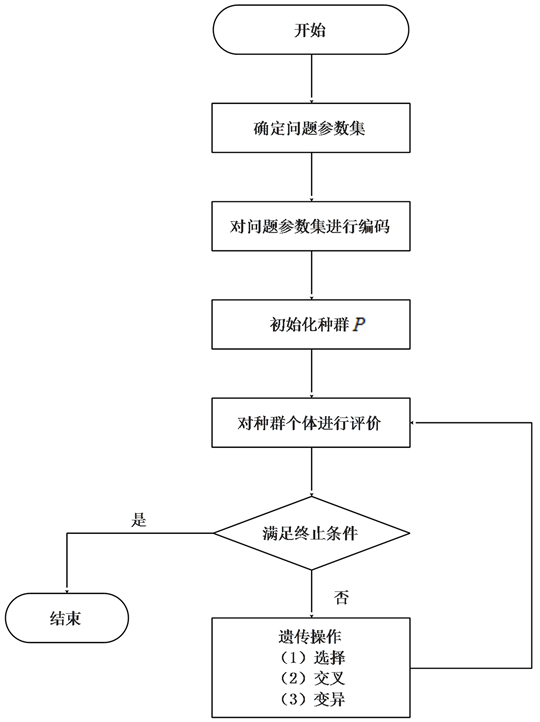Figure 2. Flow chart of genetic calculation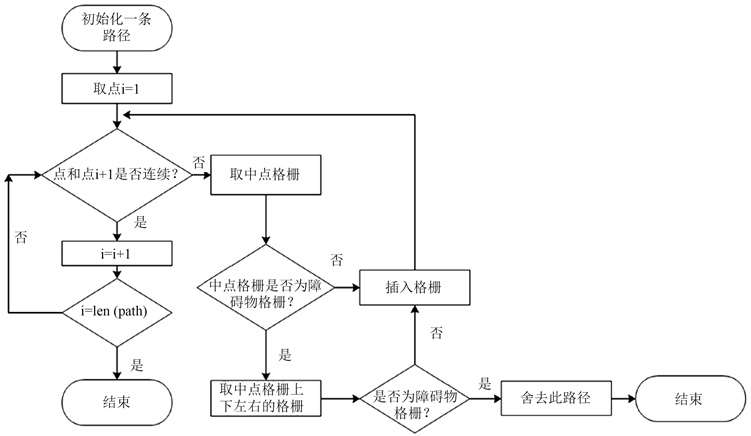Figure 3. Flow chart of initial population

3.4. 适应度函数计算

${d}_{总}\text{=}\underset{i=1}{\overset{end-1}{\sum }}\sqrt{{\left({x}_{i+1}-{x}_{i}\right)}^{2}+{\left({y}_{i+1}-{y}_{i}\right)}^{2}}$

$fit=1/{d}_{总}$

${d}_{3}=\underset{i=1}{\overset{end-2}{\sum }}\sqrt{{\left({x}_{i+2}-x\right)}^{2}+{\left({y}_{i+2}-{y}_{i}\right)}^{2}}$

d3的值可对应路径中连续三点的夹角的四种情况分别为180度、钝角、直角和锐角，其中180度的三点成一直线，平滑度最好，钝角、直角次之。由于飞行器动力学的约束，锐角是不允许的。所以分别对除直线外的3种情况给予惩罚，分别为5、30和无穷大，可以根据实际情况改动惩罚的大小。惩罚之和的倒数即为适应度函数的第二部分。

$fit=afi{t}_{1}+bfi{t}_{2}$

3.5. 选择方法

${p}_{i}=fit/\underset{i=1}{\overset{end}{\sum }}fi{t}_{i}$

3.6. 交叉方式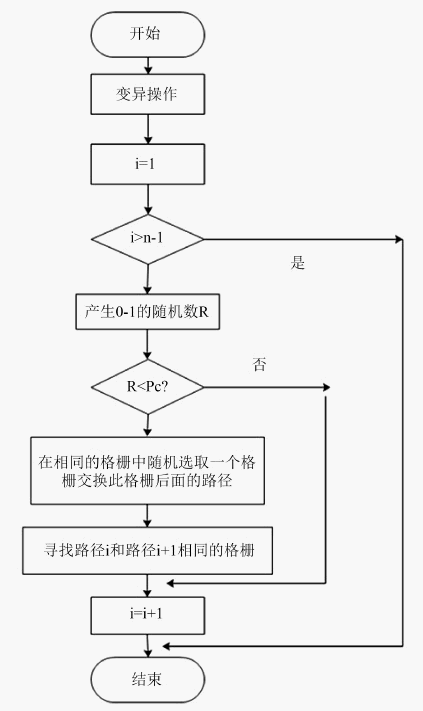Figure 4. Flow chart of crossover method

3.7. 变异方式Figure 5. Flow chart of crossover method

4. 仿真结果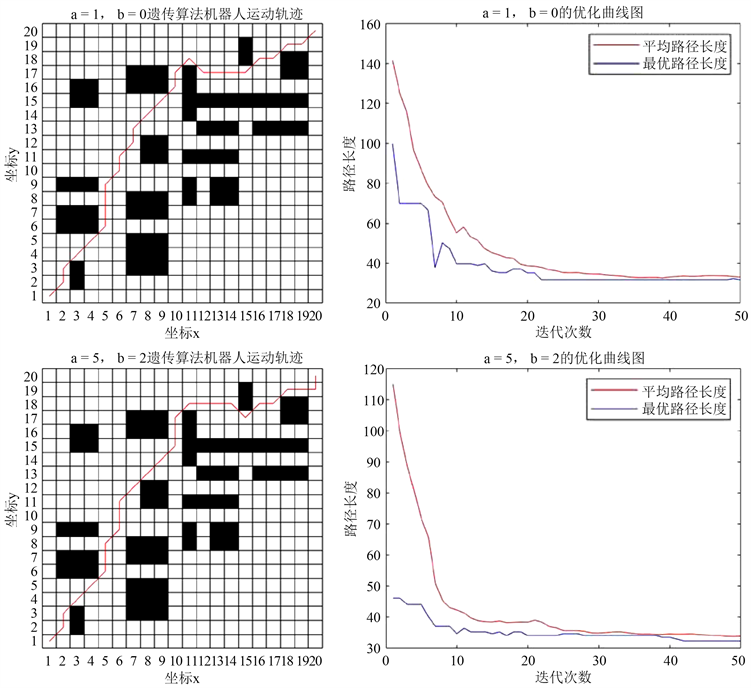Figure 6. Results of genetic algorithm

5. 创新特色

6. 结论

 王振华, 章卫国. 基于改进概率地图的无人机实时避障研究[J]. 计算机工程与应用, 2010, 46(25): 220-222.

 周炜, 魏瑞轩, 董志兴. 基于层次分解策略无人机编队避障方法[J]. 系统工程与电子技术, 2009, 31(5): 1152-1157.

 张跃东, 李丽, 刘晓波, 邢泽平. 基于单目视觉的无人机障碍探测算法研究[J]. 激光与红外, 2009, 39(6): 673-676.

 朱灿杰, 曹鑫扬, 付豪. 基于SLAM技术的智能避障无人机[J]. 科技风, 2019(13): 72.

 成浩浩, 杨森, 齐晓慧. 面向城市环境的四旋翼无人机在线避障航迹规划方法[J]. 计算机科学, 2019, 46(4): 241-246.

 于建均, 赵少琼, 郑逸加, 阮晓钢, 吴鹏申. 基于模糊专家决策的室内无人机避障系统[J]. 控制工程, 2019, 26(3): 423-430.

 杨磊, 陈凤翔, 陈科羽, 刘胜南. 基于多传感器的无人机避障方法研究及应用[J]. 计算机测量与控制, 2019, 27(1): 280-283+287.

 王海群, 王水满, 张怡. 基于激光雷达信息的无人机避障控制研究[J/OL]. 激光杂志: 1-5[2019-07-13]. http://kns.cnki.net/kcms/detail/50.1085.tn.20190508.1516.014.html

Top